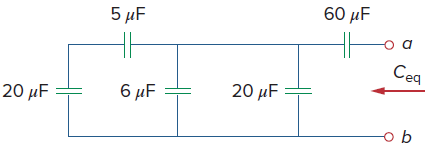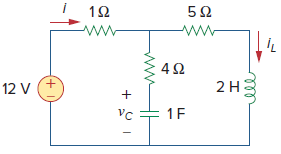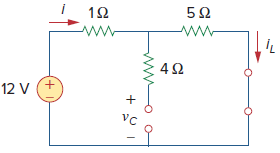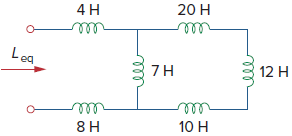# SSC JE Electrical Important MCQ Part 17

SSC JE Electrical Important MCQ PDF Part 17

1.Equivalent capacitance seen at the terminals of the circuit in Fig. isA.20 μF
B.40 μF
C.60 μF
D.30 μF

(The 20-μF and 5-μF capacitors are in series; their equivalent capacitance is=20×5/20+5== 4 μF
This 4-μF capacitor is in parallel with the 6-μF and 20-μF capacitors;their combined capacitance is 4 + 6 + 20 = 30 μF
This 30-μF capacitor is in series with the 60-μF capacitor. Hence, the equivalent capacitance for the entire circuit is
Ceq =30×60/30 + 60= 20 μF

2.Consider the circuit in Fig.Under dc conditions,The value of iL isA.9 A
B.4 A
C.6 A
D.2 A

(Under dc conditions, we replace the capacitor with an open circuit and the inductor with a short circuit, as in Fig. belowi = iL =12/1 + 5= 2 A )

3.The equivalent inductance of the circuit shown in Fig isA.42 H
B.6 H
C.18 H
D.10 H

(The 10-H, 12-H, and 20-H inductors are in series=10+12+20= 42 H
This 42-H inductor is in parallel with the 7-H inductor=7× 42/7 + 4== 6 H
This 6-H inductor is in series with the 4-H and 8-H inductors. Hence,Leq = 4 + 6 + 8 = 18 H)

4.A transistor has a collector current of 10 mA and a base current of 40 μA. What is the current gain of the transistor

A.250
B.300
C.360
D.280

Answer  : A (Divide the collector current by the base current to get βdc=Ic/Ib=10 mA/40 μA=250)

5.What is the capacity of a battery which can deliver a current of 20 Amps continuously for 2 hours and 30 minutes

A.50 AH
B.46 AH
C.40 AH
D.60 AH

Answer  : A (Battery Capacity= Amps * hours=20*150/60=50 AH)

6.The series field of a compound generator is wound with

A.few turns in thick wire
B.few turns in thin wire
C.more tuns in thick wire
D.more turns in thin wire

Answer  : A (In a Compound Wound Generator, there are two sets of the field winding on each pole. One of them is connected in series having few turns of thick wire, and the other is connected in parallel having many turns of fine wire with the armature winding)

7.The unit of electric flux density is

B.Coulomb/sq.meter
C.Coulomb
D.Weber/sq.meter

Answer  : B (D=φ/A=Q/4πr²,The SI unit of electric flux is Coulomb per meter square.)

8.The full form of ELCB is

A.Earth live circuit breaker
B.Earth leakage current breaker
C.Earth leakage circuit breaker
D.Earth line current breaker

9.Which type of insulators are used on 132 kV transmission lines

A.Pin type
B.Pin and Shackle type
C.Disc type
D.Shackle type

Answer  : C (The suspension insulator is called as Disc Insulator,Use for 11 kV to 765 kV)

10.What is the purpose of pole shoes in DC generator

A.Magnetise the poles and produce the magne tic flux
B.Provide the mechanical support to magnetic poles
C.Provide the low resistance path to eddy currents
D.Spread out the magnetic flux uniformly in the air gap

11.As per the IE rules, the maximum load on the light and fan sub-circuit should be restricted to

A.500 Watts
B.1000 Watts
C.650 Watts
D.800 Watts

Answer  : D (As per Indian Standard Specification (I.S.S), each lighting sub-circuit must have ten number of points or 800 watt whichever is less)

12.What is the name of the power generating station which uses the potential energy of water

A.Hydro power station
B.Thermal power station
C.Atomic power station
D.Nuclear power station

13.If two inductors of 7mH and 3mH are connected in series across the 250V 50Hz AC supply then the total inductive reactance will be

A.31.42 Ohms
B.10 Ohms
C.21 Ohms
D.3.142 Ohms

(Total Reactance is given by X=X₁+X₂=ω(L₁+L₂)=2πf(L₁+L₂)=2×3.14×50(7mH+3mH)=3.14×100(10×10⁻³)=3.14)

14.Type of DC motor used in electrical traction is

A.DC Series motor
B.DC Cummulative compound motor
C.DC Cummulative long shunt motor
D.All of these

Answer  : A ( The dc series motor develops high torque at start which is an essential requirement of traction service)

15.When the 3 phase power is measured by two wattmeter method, at what power factor, the readings of both wattmeter will be equal and positive

A.Unity
B.0.5 Lag
C.Zero Power
D.Less than 0.5 Lag

(PF=unity, So φ= 0

W1= VLI Cos(30° – 0)= VLI Cos30°= 0.866 VLI

W2= VLI Cos(30° + 0) = VLI Cos30°= 0.866 VLI

Thus when the power factor of load is unity then both the wattmeter reads the same value)

16.The chopper circuit converts __________ voltage

A.Fixed DC to variable AC
B.Fixed AC to variable DC
C.Fixed AC to variable AC
D.Fixed DC to variable DC

Answer  : D (A chopper is a device that converts fixed DC input to a variable DC output voltage directly)

17.The time required by an AC quantity to complete one cycle is

A.power factor
B.frequency
C.cycle/ sec
D.period

18.In controlled rectifier ________ device is used

A.Diode
B.SCR
C.Snubber
D.TRIAC

19.For protection of three phase induction motor from single phasing, ________ is used.

B.Air break circuit breaker
C.Air blast circuit breaker
D.Bucholtz relay

Answer  : A (A single phasing fault on an Induction Motor will cause the current in the other two phases to rise. To prevent damage to the motor winding, an over current protection relay/Electromagnetic over load device is normally fitted in the motor starter circuit)

20.In single phase AC circuit with impedance Z and resistance R, power factor is given by

A.Z/R
B.R/Z
C.Cos(R/Z)
D.Sin(Z/R)